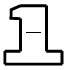### Home > CC2MN > Chapter 4 > Lesson 4.4.1 > Problem4-131

4-131.

Find the numbers that belong in the Giant One in each problem to create an equivalent fraction. Copy each problem, and show the values you used within the Giant One.

1. $\frac{7}{12}\cdot$$=\frac{56}{m}$

$7(?)=56$

$\frac{8}{8}$

1. $\frac{8}{3}\cdot$$=\frac{n}{48}$

$3(?)=48$

$\frac{16}{16}$

1. $\frac{20}{26}\cdot$$=\frac{k}{39}$

$26(?)=39$

1. $\frac{20}{42}\cdot$$=\frac{50}{h}$

$20(?)=50$